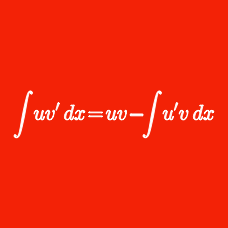Calculus

# Integration Techniques: Level 2 Challenges

$\large \int_{-\frac \pi 2 }^{\frac \pi 2 } \bigg [ e^{ \sin(x)} \cos (x )\bigg ] \ dx = a - \frac 1 a, \ \ \ a > 0, \ \ \ \ \ a = \ ?$

$\large\int_{1}^{a}\dfrac{x-1}{x+\sqrt{x}}dx=4$

Let $$a>1$$ be a constant satisfying the equation above. What is the value of $$\large a^2+a+1$$?

Evaluate the following integral

$\large\displaystyle \int \dfrac{\sin(x)}{\cos^3(x)} \, \Bbb{d}x$

$\int_0^2 x \sqrt{1-(x-1)^2} \, dx = \, ?$

Give your answer to 2 decimal places.

Evaluate the indefinite integral below.

$\large \int \frac{dx}{1 + e^{-x}}$

×

Problem Loading...

Note Loading...

Set Loading...